## 转：EXCEL一对多条件查找显示多个结果（INDEX+SMALL+IF+ROW函数组合）

2021-07-14 浏览:1655【INDEX+SMALL+IF+ROW】组合函数：

①难度：★★★☆☆
②作用：实现查找时返回多个符合条件的结果。
③结果放在行的写法：

`INDEX(结果列,SMALL(IF(条件,ROW(条件列),4^8),COLUMN(A1)))&""`

④结果放在列的写法：

`INDEX(结果列,SMALL(IF(条件,ROW(条件列),4^8),ROW(A1)))&""`

⑤结束键：数组公式须同时按CTRL+SHIFT+ENTER三键结束。

1.原始表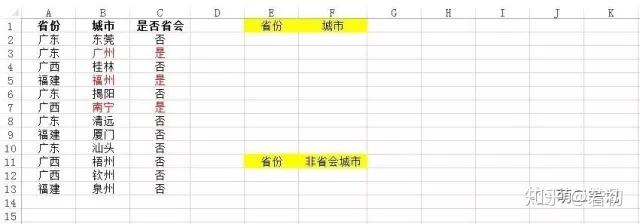2.结果表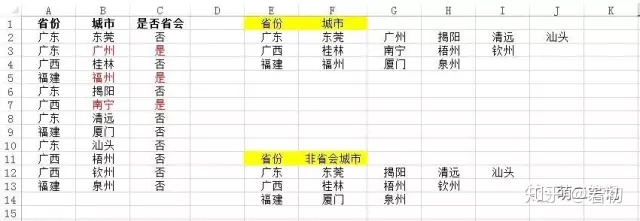3.操作过程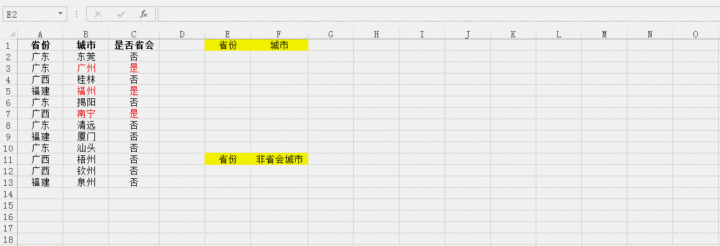4.步骤分解
①在E列提取A列省份（重复的只留一个）
方法一：复制A列粘贴到E列，EXCEL2007以上版本可直接点菜单栏“数据”，然后点击“删除重复项”，简单粗暴就OK了。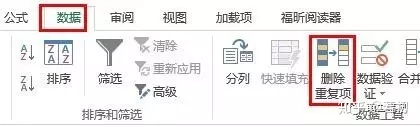方法二：INDEX+MATCH（上例用的是此法，详细的下次再探讨）

E2输入公式

`=INDEX(A:A,SMALL(IF(MATCH(\$A\$2:\$A\$13,\$A\$2:\$A\$13,0)=ROW(\$A\$2:\$A\$13)-1,ROW(\$2:\$13),4^8),ROW(1:1)))&""`

按CTRL+SHIFT+ENTER三键结束，下拉。

②在F2单元格里为E2省匹配B列中对应的第1个城市
F2输入公式

`=INDEX(\$B:\$B,SMALL(IF(\$A\$2:\$A\$13=\$E2,ROW(\$A\$2:\$A\$13),4^8),COLUMN(A1)))&""`

三键结束。

③把F2的公式右拉，分别匹配E2省对应的第2、3、4、5个城市...直到显示空白。

④把E2右边存放有公式的单元格选中后下拉，为E3、E4单元格里的省份匹配到对应的各个城市。

⑤附加题：为省份匹配非省会的城市

公式的写法同上面一样道理，只是增加了一个条件——C列等于"否"。

那就在第②点的公式里插入一个新条件

`=INDEX(\$B:\$B,SMALL(IF((\$A\$2:\$A\$13=\$E2)*(\$C\$2:\$C\$13="否"),ROW(\$A\$2:\$A\$13),4^8),COLUMN(A1)))&""`

同样三键结束。记得哦，增加条件后该增加的括号()也得成对增加哈~

⑥同理，如有更多条件要求，比照第⑤点用*星号连接插入相关条件即可。

5.公式翻译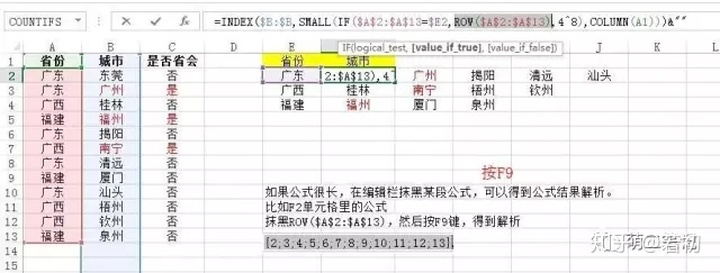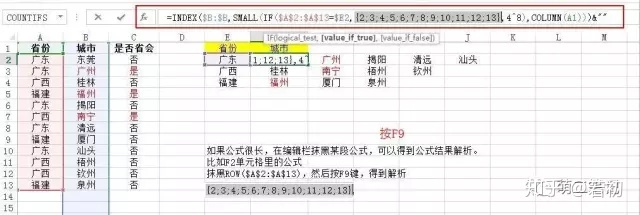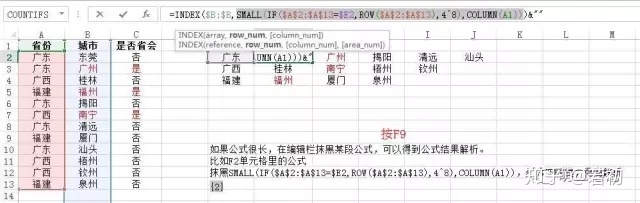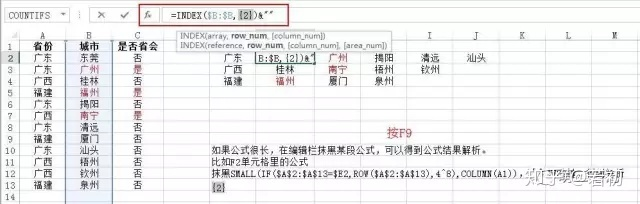`INDEX(\$B:\$B,SMALL(IF(\$A\$2:\$A\$13=\$E2,ROW(\$A\$2:\$A\$13),4^8),COLUMN(A1)))&""`

IF(A列省份若是“广东”,返回那一行的行号,不是“广东”则返回一个很大的数65536)

SMALL(IF()得到的行号数组从小到大排列,第几小的行号)

INDEX(城市列,SMALL()得到的行信息)

INDEX(城市列,A列省份是“广东”对应的第1个城市)

INDEX(城市列,A列省份是“广东”对应的第2个城市)

INDEX(城市列,A列省份是“广东”对应的第3个城市)

。。。。。。

INDEX(城市列,A列省份是“广东”对应的第N个城市)

#### 发表评论: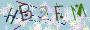◎欢迎参与讨论，请在这里发表您的看法、交流您的观点。